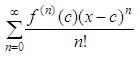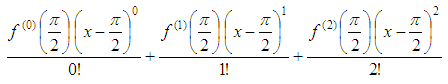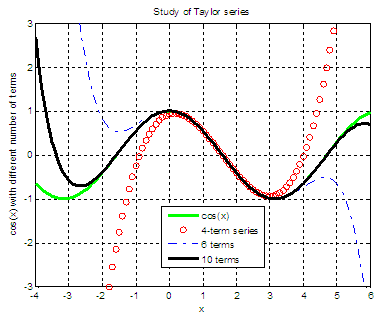# Taylor expansion - series experiments with Matlab

Once you know how Maclaurin series work, Taylor series are easier to understand.

Taylor expansions are very similar to Maclaurin expansions because Maclaurin series actually are Taylor series centered at x = 0.

Thus, a Taylor series is a more generic form of the Maclaurin series, and it can be centered at any x-value.

The goal of a Taylor expansion is to approximate function values.

You’ll have a good approximation only if you’re close to the series’ center.

Taylor series look almost identical to Maclaurin series:Note:
- The derivatives in Taylor series are evaluated at x = c, the center of the approximation,
not at x = 0.
- You raise the quantity (x – c) to the n power, not just x.

Other than that, the formulas (Maclaurin’s and Taylor’s) are identical.

As an example, let’s use a cosine series to approximate cos(1.6), using just three terms in the  Taylor polynomial for f(x) = cos(x) centered at x = pi/2
(x is approx. 1.57). In this problem, f(x) = cos(x) and c = pi/2.And we’ll need to take three derivatives (n = 0 to 2) of the function and then evaluate them at x = pi/2.

Definition 1: f(0)(x) is the function itself, in this case cos(x). It's not a derivative!
Definition 2: 0! = 1.

f(0)(x) = cos(x)   f(0)(pi/2) = cos(pi/2) = 0
f(1)(x) = -sin(x)  f(1)(pi/2) = -sin(pi/2) = -1
f(2)(x) = -cos(x)  f(2)(pi/2) = -cos(pi/2) = 0

Let’s create a fast script in Matlab to evaluate the series just with 3 terms (0 to 2):

clear, clc

% Let's see more decimals
format long

% We go from n = 0 to n = 2
n = 0 : 2;

% Center and point to evaluate
c = pi/2;
x = 1.6;

% These are the derivatives for each term
d = [0 -1 0];

% We form the sequence, following the Taylor formula
seq = d .* (x - c).^n ./(factorial(n))

% We add-up to get the Taylor approximation
approx = sum(seq)

% Let's compare with the official number
real_value = cos(x)

The resuls are:

seq =       0  -0.02920367320510                  0
approx =       -0.02920367320510
real_value =   -0.02919952230129

We’ve got almost the exact value by using only 3 terms of the series (and two values are zero because the derivative is zero for those terms!!). If we had used n = 10, it would have been an even better approximation.

Now, let’s develop an automated series to express the cosine function (centered at pi/2) using the Taylor expansion and let’s compare the results with different number of terms included.

function stp = taylor_cosine(c, x, n)
% c = center of the function
% x = a vector with values around c
% n = number of terms in the series

% Start the series with 0
smp = 0;

% Consider all the possible derivatives
deriv = [0 -1 0 1]';

% Iterate n times (from 0 to n-1)
for i = 0 : n-1

% Implement the Taylor expansion
t(i+1, :) = deriv(1) * (x-c).^(i) / factorial(i);

% Find derivative for the next term
deriv = circshift(deriv, -1);

end

% Add-up the calculated terms
stp = sum(t);

Note: the above code is not very efficient, because we’re calculating and adding terms even when their derivative is zero, which is a waste of 50% of the time, more or less. We’ll leave it as-is just to keep and show the natural flow of the formula...

Now, let’s create a script to test and use the function above.

clear, clc, close all

% Work with values around center c
c = pi/2;
x = -4 : .1 : 6;
y = cos(x);

% Plot the goal
plot(x, y, 'g', 'Linewidth', 3)
title(
'Study of Taylor series')
xlabel(
'x')
ylabel(
'cos(x) with different number of terms')
axis([-4 6 -3 3])
grid
on
hold on

% Consider 4 terms in the series
smp = taylor_cosine(c, x, 4);
plot(x, smp,
'ro')

% Consider 6 terms
smp = taylor_cosine(c, x, 6);
plot(x, smp,
'b-.')

% Consider 10 terms
smp = taylor_cosine(c, x, 10);
plot(x, smp,
'k', 'linewidth', 3)

% Label the calculated lines
legend('cos(x)', '4-term series', ...
'6 terms', '10 terms')

The results are:We see that all of the Taylor expansions work well when we are close to pi/2 (x approx. 1.57). More terms approximate better a larger portion of the cosine curve.